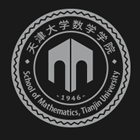﻿The computation of singular integrals and singular Volterra integral equations The Schrodinger Equation and Beyond-天津大学数学学院

# The computation of singular integrals and singular Volterra integral equations The Schrodinger Equation and Beyond

2019-09-12 08:47

This talk includes two parts.

In the first part, we consider the computation of Hadamard finite-part integrals. A general framework is constructed for efficiently and stably evaluating the Hadamard finite-part integrals by composite quadrature rules. Then a practical algorithm is designed for evaluating the Hadamard finite-part integrals by applying the Gauss-Legendre rule to the proposed framework.

In the second part, we discuss the computation of singular Volterra integral equations of the second kind. For the linear case, the Laplace transform is used to derive the asymptotic expansions for the solution about zero and infinity, which can be used to approximate the solution when the argument is small and large, respectively. For the nonlinear case, we derive the general Puiseux expansion for the solution about zero by Picard iteration and series decomposition. The expansion about zero is an accurate measurement of the singular behavior of the solution, which is indispensable to design efficiently numerical methods to solve these types of equations. We can use standard trapezoidal rule to discretize the singular integral and derive the Euler-Maclaurin asymptotic expansion using the known Puiseux expansion for the solution about zero. By accumulating some lower order error terms to the quadrature formula, we obtain high accuracy evaluations to these Volterra integral equations. The method is used to solve the Lighthill integral equation, and obtain the asymptotic expansions for the solution about zero and infinity, respectively.### 联系我们